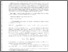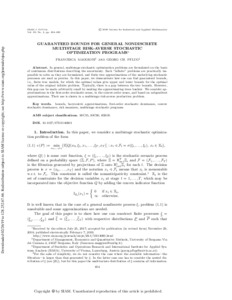# Guaranteed Bounds for General Nondiscrete Multistage Risk-Averse Stochastic Optimization Programs

Maggioni F & (2019). Guaranteed Bounds for General Nondiscrete Multistage Risk-Averse Stochastic Optimization Programs. SIAM Journal on Optimization 29 (1): 454-483. DOI:10.1137/17M1140601.Preview
Text
10.1137@17M1140601.pdf - Published Version

## Abstract

In general, multistage stochastic optimization problems are formulated on the basis of continuous distributions describing the uncertainty. Such “infinite” problems are practically impossible to solve as they are formulated, and finite tree approximations of the underlying stochastic processes are used as proxies. In this paper, we demonstrate how one can find guaranteed bounds, i.e., finite tree models, for which the optimal values give upper and lower bounds for the optimal value of the original infinite problem. Typically, there is a gap between the two bounds. However, this gap can be made arbitrarily small by making the approximating trees bushier. We consider approximations in the first-order stochastic sense, in the convex-order sense, and based on subgradient approximations. Their use is shown in a multistage risk-averse production problem.

Item Type: Article Risk & Resilience (RISK) Luke Kirwan 26 Feb 2019 14:40 26 Feb 2019 14:40 http://pure.iiasa.ac.at/15767View Item